CoCalc Shared FilesCDS-101 / Homework / HW4 / 2017Spring_cds101_hw4.html
Author: James Glasbrenner
Views : 6
Description: Jupyter html version of CDS-101/Homework/HW4/2017Spring_cds101_hw4.ipynb
(File too big to render with math typesetting.)
2017Spring_cds101_hw4

# Homework 4¶

**CDS-101 (Spring 2017)**

**Name:** Helena Gray

The code below loads the necessary packages for data analysis and reads in the datasets 'starbucks.csv' and 'simdata.RData'.

In :
# Set the location of our personally installed R packages
.libPaths(new = "~/Rlibs")
# Load the Tidyverse and modelr packages
library(tidyverse)
library(modelr)
# Provide notification if NA values are dropped when modeling
options(na.action = na.warn)
# Load the datasets for Homework 4

## Uncomment the code below to change the plot window size
# options(repr.plot.width = 6, repr.plot.height = 4)

## Uncomment the code below to change the tibble printing format.
# options(tibble.print_max = 30, tibble.print_min = 20,
#         tibble.width = Inf)

Parsed with column specification:
cols(
item = col_character(),
calories = col_integer(),
fat = col_double(),
carb = col_integer(),
fiber = col_integer(),
protein = col_integer(),
type = col_character()
)


### Question 1.¶

Based on my knowledge, the amount of carbohydrates a food item contains is positively correlated with how many calories that food item provides. In this case, the number of calories will be the explanatory variable and the response variable will be the number of carbohydrates. This model is probably not true for all amounts of calories. Some foods have high amounts of fat and sugar but low amounts of carbohydrates and still provide a high number of calories.

### Question 2.¶

The code below makes use of a couple of functions to model the linear relationship between carbohydrate content and calorie content. The code uses the lm() function to model the 'carb' and 'calories' columns from the 'starbucks' dataset to create a visual representation to describe the relationship between the two variables. The data_grid() function creates a tibble that has a column of each unique value of the specified column, in this case it's 'calories'. The add_predictions() function adds the predicted values of the response variable to the tibble containing the unique values of the explanatory variable. ggplot() and geom_point() plot the actual values of the 'calories' and 'carbs' columns while the geom_line() function takes the predicted response variable values and plots a line to illustrate the trend of the relationship between calories and carbohydrates.

In :
sim1_model <- lm(carb~calories, starbucks)
sim1_model

grid <- data_grid(starbucks, calories)
print(grid)

print(grid)

ggplot(starbucks) +
geom_point(aes(calories, carb)) +
geom_line(aes(calories, pred), data=grid, color="red", size=1)

Call:
lm(formula = carb ~ calories, data = starbucks)

Coefficients:
(Intercept)     calories
8.944        0.106

# A tibble: 29 × 1
calories
<int>
1        80
2       130
3       140
4       160
5       170
6       180
7       190
8       210
9       270
10      280
# ... with 19 more rows
# A tibble: 29 × 2
calories     pred
<int>    <dbl>
1        80 17.42603
2       130 22.72758
3       140 23.78788
4       160 25.90850
5       170 26.96881
6       180 28.02912
7       190 29.08943
8       210 31.21005
9       270 37.57190
10      280 38.63221
# ... with 19 more rows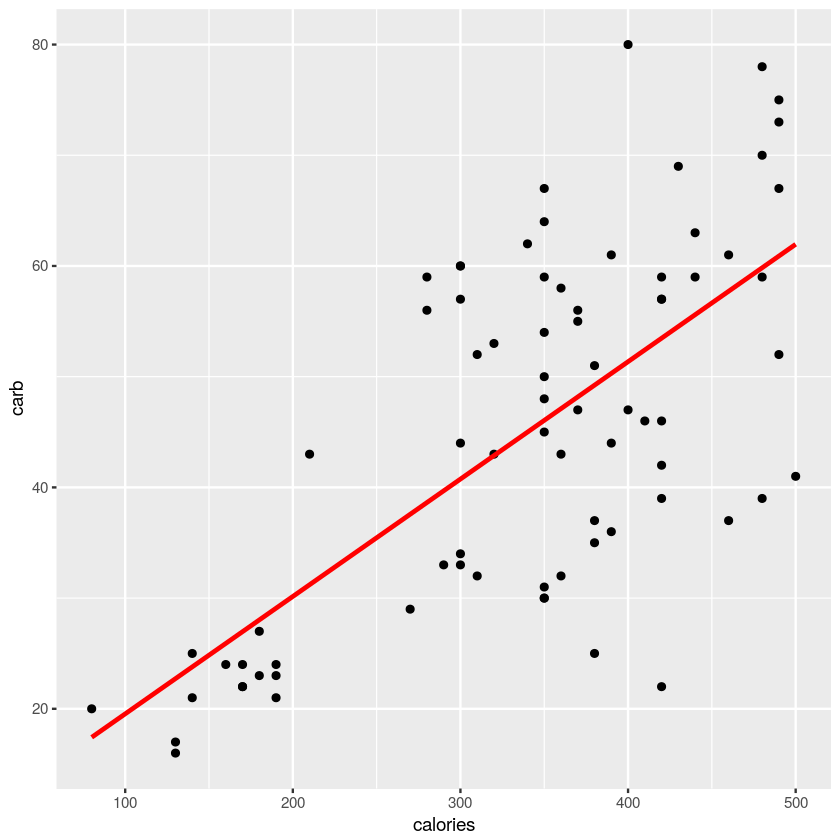The add_residuals() function adds the residuals to the tibble, which are the differences between the predicted response variable values and the actual response variable values for each unique explanatory variable value. The geom_ref_line() function creates the thick white line as the reference line that symbolizes no difference between predicted and actual values. ggplot() and geom_point() maps out the residual points for each unique value of calories.

In :
sim1_resid <- add_residuals(starbucks, sim1_model)

ggplot(sim1_resid) +
geom_ref_line(h=0) +
geom_point(aes(calories, y=resid))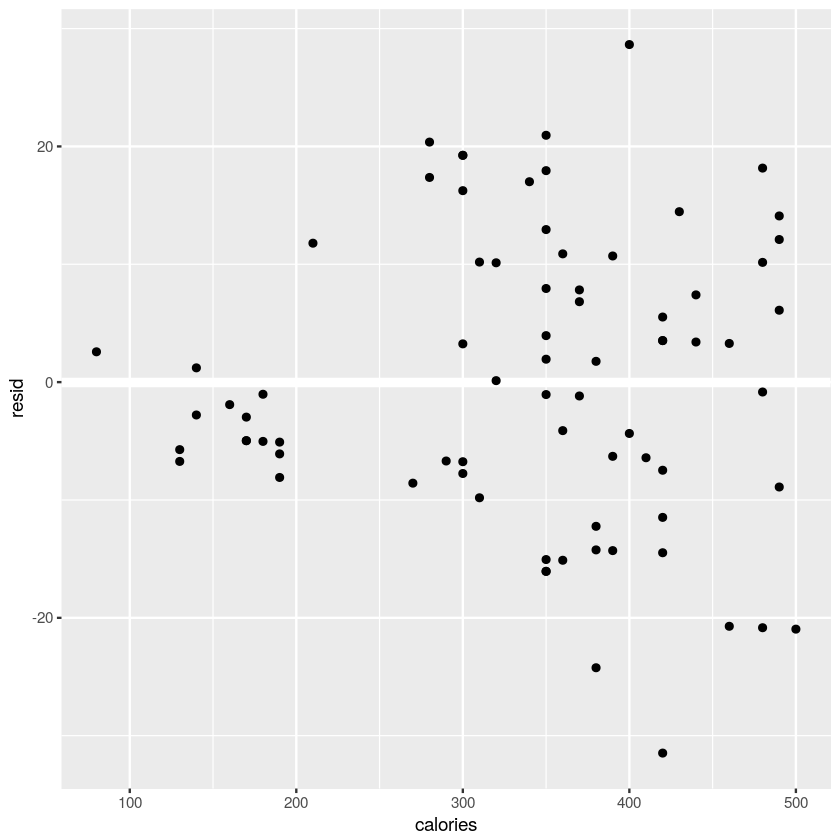It seems that food items with a low amount of calories have less variation in the discrepancy between actual and predicted values of carbohydrates while those food items with higher numbers of calories have greater variation.

The code below creates a frequency polygon plot of the residuals using ggplot() and geom_freqpoly() functions. It's like a line graph version of a histogram.

In :
ggplot(sim1_resid) +
geom_freqpoly(aes(resid), binwidth=0.5)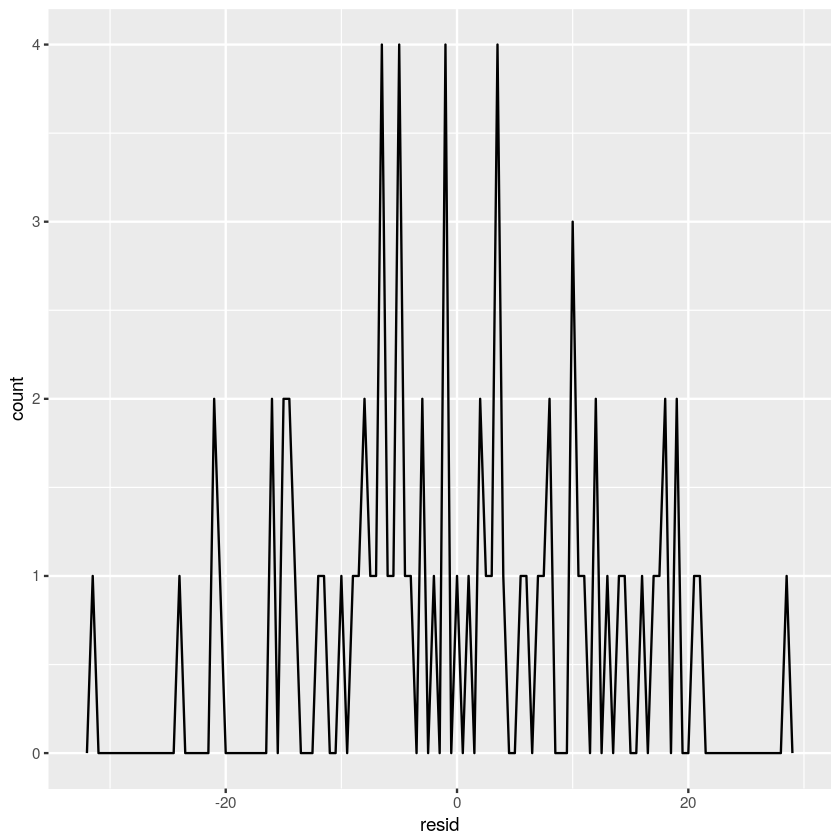The distribution of residuals appears to be relatively normal, with most of the residuals being around 0 (indicating the linear model is fairly accurate).

### Question 3.¶

This data seems to meet the required conditions for a least squares regression line as the data is relatively linear (1), has a nearly normal distribution of residuals (2), and the residuals of a linear regression have a constant variance because they are by definition random (3).

### Question 4.¶

The code below separates the simdata dataset to three different tibbles for each simulated dataset by using the filter() function and specifying the label of the simulated dataset.

In :
sim_a_simdata<-filter(simdata, label=='sim_a')

sim_b_simdata<-filter(simdata, label=='sim_b')

sim_c_simdata<-filter(simdata, label=='sim_c')


The code below fits a linear model to the sim_a dataset, plots the linear model and the dataset to demonstrate how well the lienar model represents the dataset, and then plots the residuals in a scatterplot and a frequency polygon plot to show if the variability of the residuals is constant which is one of the conditions necessary to satisfy to justify the use of a linear model.

In :
sim_a_model <- lm(y~x, sim_a_simdata)
sim_a_model

grid <- data_grid(sim_a_simdata, x)
print(grid)

print(sim_a_resid)

print(grid)

sima_plot<-ggplot(sim_a_simdata) +
geom_point(aes(x, y)) +
geom_line(aes(x, y=pred), data=grid, color="red", size=1) +
geom_abline(intercept=6,slope=1.5)

sima_plot

sima_resid_scatterplot<-ggplot(sim_a_resid) +
geom_ref_line(h=0) +
geom_point(aes(x, y=resid))

sima_resid_scatterplot

sima_freqpoly<-ggplot(sim_a_resid) +
geom_freqpoly(aes(resid), binwidth=0.5)

sima_freqpoly

Call:
lm(formula = y ~ x, data = sim_a_simdata)

Coefficients:
(Intercept)            x
6.153        1.482

# A tibble: 10 × 1
x
<int>
1      1
2      2
3      3
4      4
5      5
6      6
7      7
8      8
9      9
10    10
# A tibble: 30 × 4
x         y label      resid
<int>     <dbl> <chr>      <dbl>
1      1  6.664979 sim_a -0.9696820
2      1  7.982189 sim_a  0.3475283
3      1  8.304699 sim_a  0.6700386
4      2  6.259045 sim_a -2.8574711
5      2  8.606901 sim_a -0.5096155
6      2  6.221735 sim_a -2.8947809
7      3 11.315656 sim_a  0.7172836
8      3 11.454724 sim_a  0.8563517
9      3  9.895552 sim_a -0.7028202
10     4 12.648988 sim_a  0.5687603
# ... with 20 more rows
# A tibble: 10 × 2
x      pred
<int>     <dbl>
1      1  7.634661
2      2  9.116516
3      3 10.598372
4      4 12.080228
5      5 13.562083
6      6 15.043939
7      7 16.525795
8      8 18.007651
9      9 19.489506
10    10 20.971362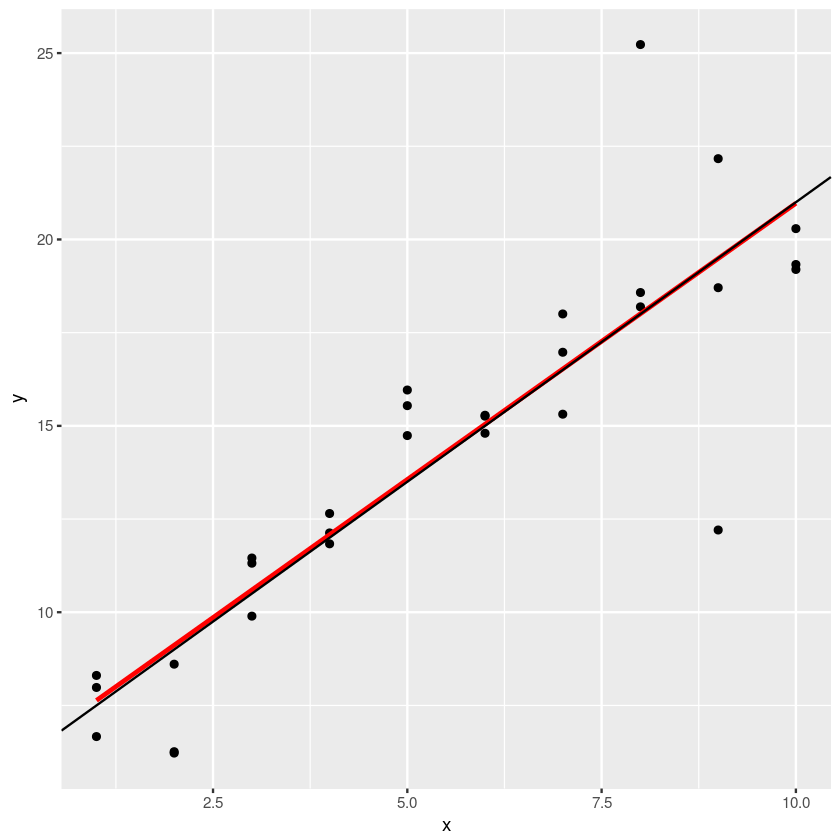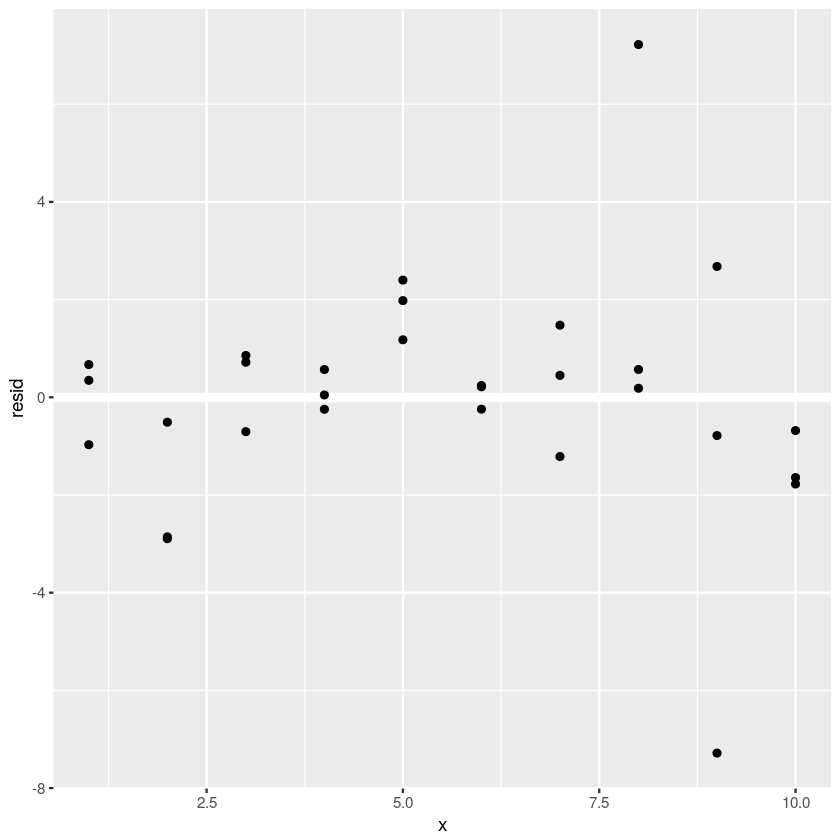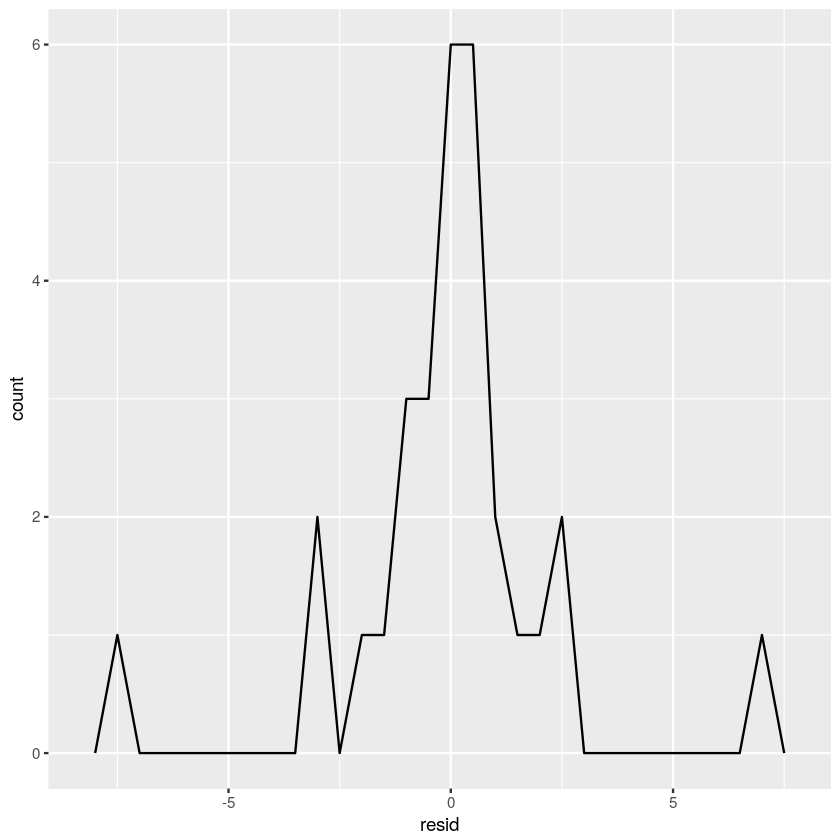This dataset seems to conform pretty well to the linear model and has a fairly normal distribution of residuals and constant variance.

The code below fits a linear model to the sim_b dataset, plots the linear model and the dataset to demonstrate how well the lienar model represents the dataset, and then plots the residuals in a scatterplot and a frequency polygon plot to show if the variability of the residuals is constant which is one of the conditions necessary to satisfy to justify the use of a linear model.

In :
sim_b_model <- lm(y~x, sim_b_simdata)
sim_b_model

grid <- data_grid(sim_b_simdata, x)
print(grid)

print(sim_b_resid)

print(grid)

simb_plot<-ggplot(sim_b_simdata) +
geom_point(aes(x, y)) +
geom_line(aes(x, y=pred), data=grid, color="red", size=1) +
geom_abline(intercept=6,slope=1.5)

simb_plot

simb_resid_scatterplot<-ggplot(sim_b_resid) +
geom_ref_line(h=0) +
geom_point(aes(x, y=resid))

simb_resid_scatterplot

simb_freqpoly<-ggplot(sim_b_resid) +
geom_freqpoly(aes(resid), binwidth=0.5)

simb_freqpoly

Call:
lm(formula = y ~ x, data = sim_b_simdata)

Coefficients:
(Intercept)            x
5.748        1.498

# A tibble: 10 × 1
x
<int>
1      1
2      2
3      3
4      4
5      5
6      6
7      7
8      8
9      9
10    10
# A tibble: 30 × 4
x         y label      resid
<int>     <dbl> <chr>      <dbl>
1      1  6.463563 sim_b -0.7822471
2      1  6.895462 sim_b -0.3503476
3      1  6.497586 sim_b -0.7482242
4      2  9.740443 sim_b  0.9967212
5      2  9.440358 sim_b  0.6966354
6      2  5.749456 sim_b -2.9942662
7      3 12.260701 sim_b  2.0190666
8      3 12.666744 sim_b  2.4251097
9      3  7.124891 sim_b -3.1167432
10     4 13.690909 sim_b  1.9513625
# ... with 20 more rows
# A tibble: 10 × 2
x      pred
<int>     <dbl>
1      1  7.245810
2      2  8.743722
3      3 10.241635
4      4 11.739547
5      5 13.237459
6      6 14.735372
7      7 16.233284
8      8 17.731197
9      9 19.229109
10    10 20.727021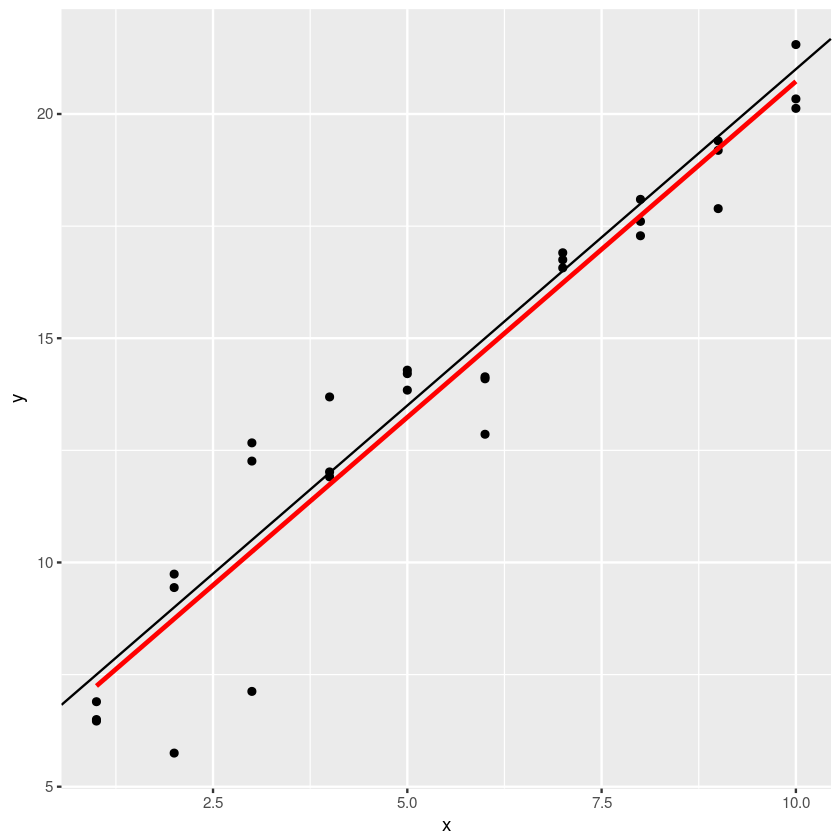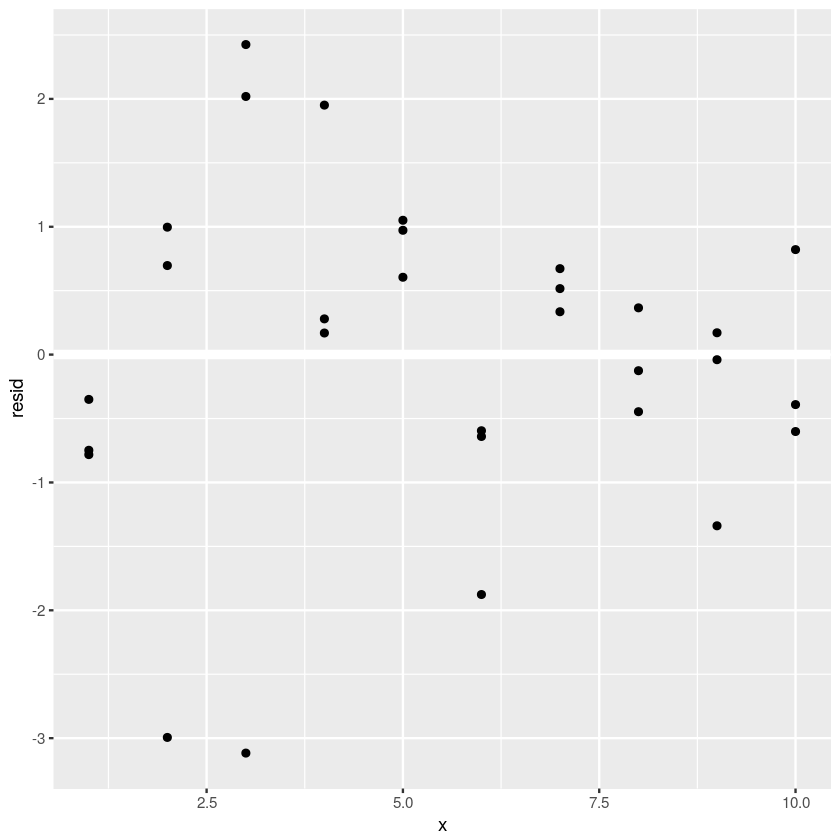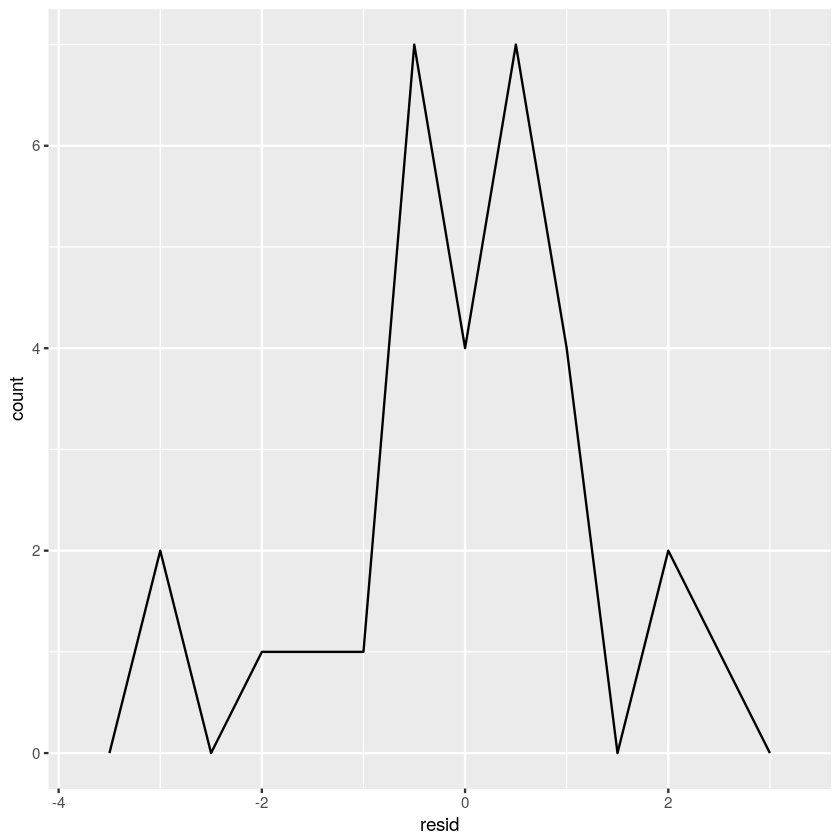This linear model seems to have a relatively normal distribution of residuals and constant variance however the scatterplot of residuals reveals that the higher values of x seem to conform more closely to the linear model than the lower values of x indicating that there may be another type of correlation that more accurately represents the relationship between x and y than a linear correlation.

The code below fits a linear model to the sim_c dataset, plots the linear model and the dataset to demonstrate how well the lienar model represents the dataset, and then plots the residuals in a scatterplot and a frequency polygon plot to show if the variability of the residuals is constant which is one of the conditions necessary to satisfy to justify the use of a linear model.

In :
sim_c_model <- lm(y~x, sim_c_simdata)
sim_c_model

grid <- data_grid(sim_c_simdata, x)
print(grid)

print(sim_c_resid)

print(grid)

simc_plot<-ggplot(sim_c_simdata) +
geom_point(aes(x, y)) +
geom_line(aes(x, y=pred), data=grid, color="red", size=1) +
geom_abline(intercept=6,slope=1.5)

simc_plot

simc_resid_scatterplot<-ggplot(sim_c_resid) +
geom_ref_line(h=0) +
geom_point(aes(x, y=resid))

simb_resid_scatterplot

simc_freqpoly<-ggplot(sim_c_resid) +
geom_freqpoly(aes(resid), binwidth=0.5)

simc_freqpoly

Call:
lm(formula = y ~ x, data = sim_c_simdata)

Coefficients:
(Intercept)            x
6.201        1.494

# A tibble: 10 × 1
x
<int>
1      1
2      2
3      3
4      4
5      5
6      6
7      7
8      8
9      9
10    10
# A tibble: 30 × 4
x         y label       resid
<int>     <dbl> <chr>       <dbl>
1      1  7.269889 sim_c -0.42554092
2      1  8.156796 sim_c  0.46136619
3      1  7.642158 sim_c -0.05327174
4      2  9.276237 sim_c  0.08640271
5      2  9.295213 sim_c  0.10537924
6      2  8.669480 sim_c -0.52035362
7      3 12.656824 sim_c  1.97258569
8      3 11.296132 sim_c  0.61189401
9      3 10.306466 sim_c -0.37777264
10     4 12.461735 sim_c  0.28309287
# ... with 20 more rows
# A tibble: 10 × 2
x      pred
<int>     <dbl>
1      1  7.695430
2      2  9.189834
3      3 10.684238
4      4 12.178642
5      5 13.673047
6      6 15.167451
7      7 16.661855
8      8 18.156259
9      9 19.650663
10    10 21.145067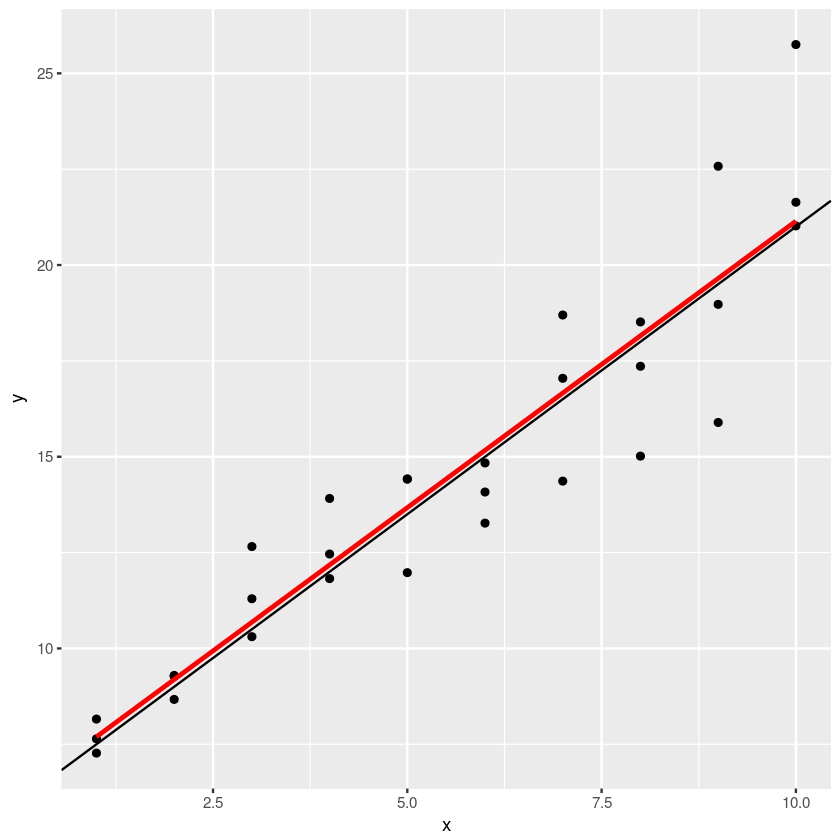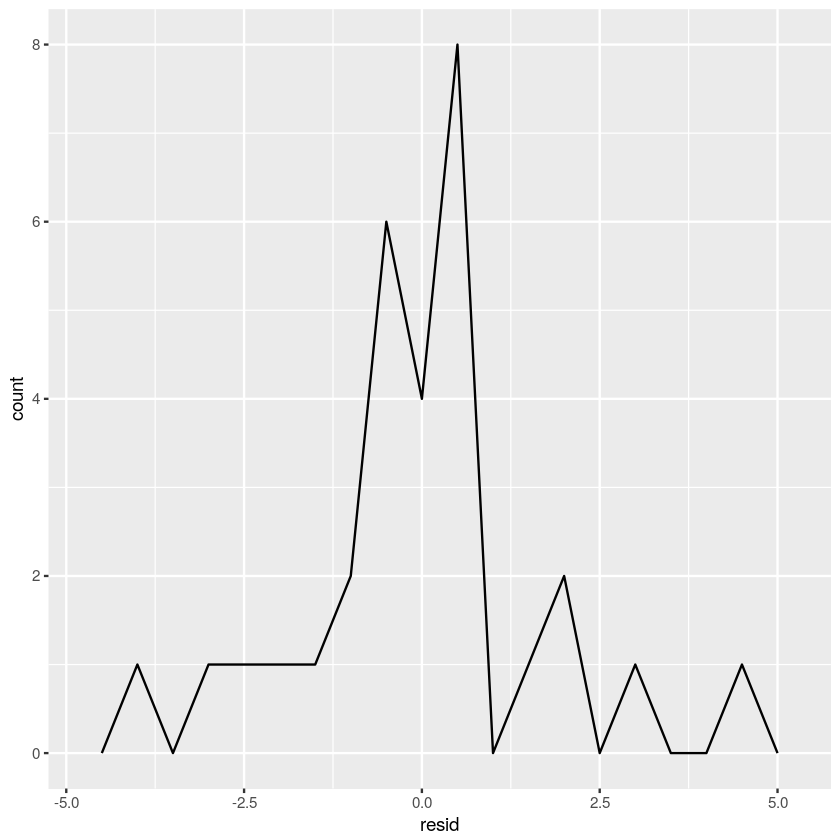In [ ]:



### Question 5¶

The following code shows an alternative to the least-squares criterion, the mean-absolute distance criterion, which involves averaging over the absolute value of residuals instead of squaring them. This is implemented by using the function optim() in combination with the custom function 'make_prediction' shown below.

The code uses the optim() function to fit the "sim_a", "sim_b", and "sim_c" datasets from simdata using the mean absolute distance. The plot for the sim_a dataset shows both the mean-absolute distance and least-squares lines.

In :
make_prediction <- function(intercept_slope, data)
{
intercept_slope + data$x * intercept_slope } measure_distance <- function(intercept_slope_parameters, dataset) { diff <- data$y - make_prediction(intercept_slope_parameters,
dataset)
mean(abs(diff))
}

In :
measure_distance(c(6.153,1.482), sim_a_simdata)

sima_model_parameters <- optim(c(16, -5), measure_distance, data=sim_a_simdata)
print(sima_model_parameters$par) ggplot(sim_a_simdata) + geom_point(aes(x,y),size=2, color="grey30") + geom_line(aes(x, pred), data=grid, color="red", size=1) + geom_abline(intercept=sima_model_parameters$par,slope=sima_model_parameters$par)  Error in data$y: object of type 'closure' is not subsettable
Traceback:

1. measure_distance(c(6.153, 1.482), sim_a_simdata)
In [ ]:
measure_distance(c(2,0.7), sim_b_simdata)

simb_model_parameters <- optim(c(16, -5), measure_distance, data=sim_b_simdata)
print(simb_model_parameters$par) ggplot(sim_b_simdata, aes(x, y)) + geom_point(size=2, color="grey30") + geom_abline(intercept=simb_model_parameters$par,
slope=simb_model_parameters\$par)


### Question 5¶

Another alternative to lm() is to fit a smooth curve using the loess() function. Follow the procedure in Chapter 23.3 of R for Data Science of model fitting, grid generation, predictions, and visualization on the sim1 dataset (loaded as part of the modelr library). The plot should also include the least-squares line for comparison. Calculate the residuals and frequency polygon. How does this compare with the least-squares line? How does it compare with the default method of geom_smooth()?

In [ ]:
sim1_mod <- loess(y ~ x, data = sim1)
sim1_mod

grid <- sim1 %>%
data_grid(x)

grid <- grid %>%

ggplot(sim1, aes(x)) +
geom_point(aes(y = y)) +
geom_line(aes(y = pred), data = grid, colour = "red", size = 1)

sim1 <- sim1 %>%

ggplot(sim1, aes(resid)) +
geom_freqpoly(binwidth = 0.5)

ggplot(sim1, aes(x, resid)) +
geom_ref_line(h = 0) +
geom_point()

In [ ]:
ggplot(sim1) +
geom_point(aes(x=x, y = y)) +
geom_smooth(mapping=aes(x=x,y=y),method='lm',se=FALSE)

In [ ]:


;